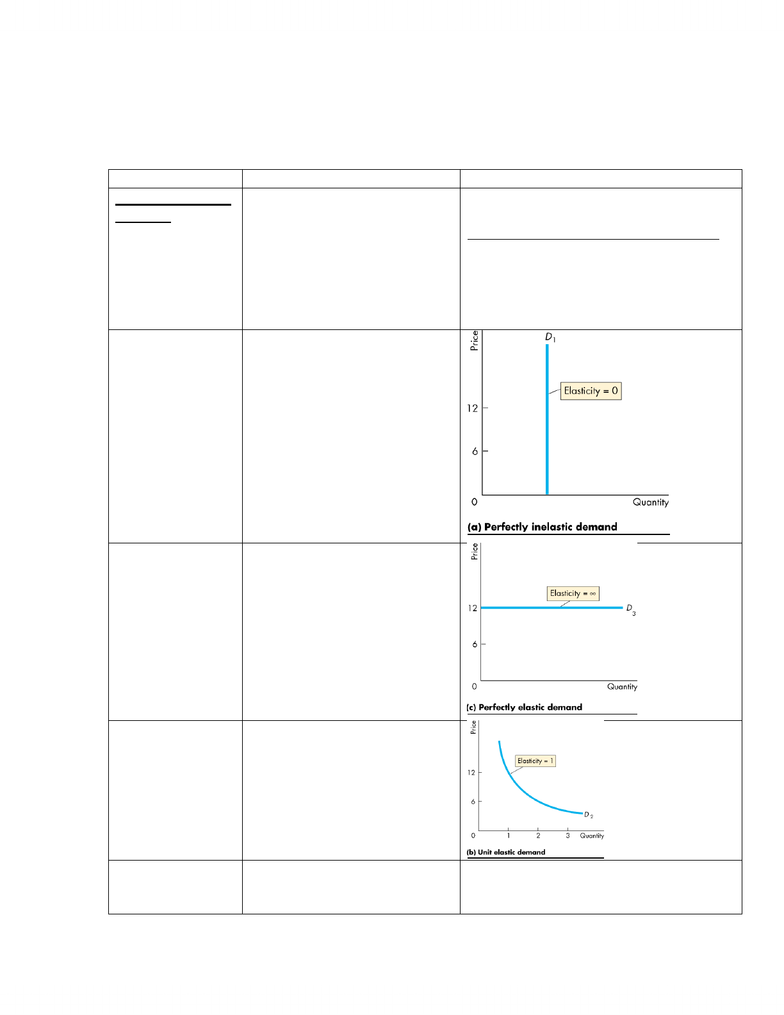# Economics 1021A/B Lecture Notes - Test Method

23 views4 pages24 Apr 2012
School
DepartmentNicole Wallenburg
Mr. Parkin
Economics
Oct 3, 2011
Economics Lecture #7
Definition
Info
Price Elasticity of
Demand
The price elasticity of demand
is a units-free measure of the
responsiveness of the quantity
demanded of a good to a change
in its price when all other
remain the same.
Formula:
Percentage change in quantity demanded
Percentage change in price
Inelastic
If the quantity demanded doesn’t
change when the price changes,
the price elasticity of demand is
zero and the good has a
perfectly inelastic demand.
If the percentage change in the
quantity demanded is smaller
than the percentage change in
price, the price elasticity of
demand is less than 1 and the
good has inelastic demand.
Elastic Demand
If the percentage change in the
quantity demanded is greater
than the percentage change in
price the price elasticity of
demand is greater than 1 and the
good has elastic demand.
If the price elasticity of demand
is infinity, the good has
perfectly elastic demand.
Unit Elastic
If the percentage change in the
quantity demanded equals the
percentage change in price, the
price elasticity of demand equals
1 and the good has unit elastic
demand
Total Revenue
and Elasticity
The total revenue from the sale
of good or service equals the
price of the good multiplied by
The change in total revenue due to a
change in price depends on the elasticity of
demand:
Unlock document

This preview shows page 1 of the document.
Unlock all 4 pages and 3 million more documents.

# Get access

\$10 USD/m
Billed \$120 USD annually
Homework Help
Class Notes
Textbook Notes